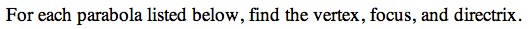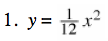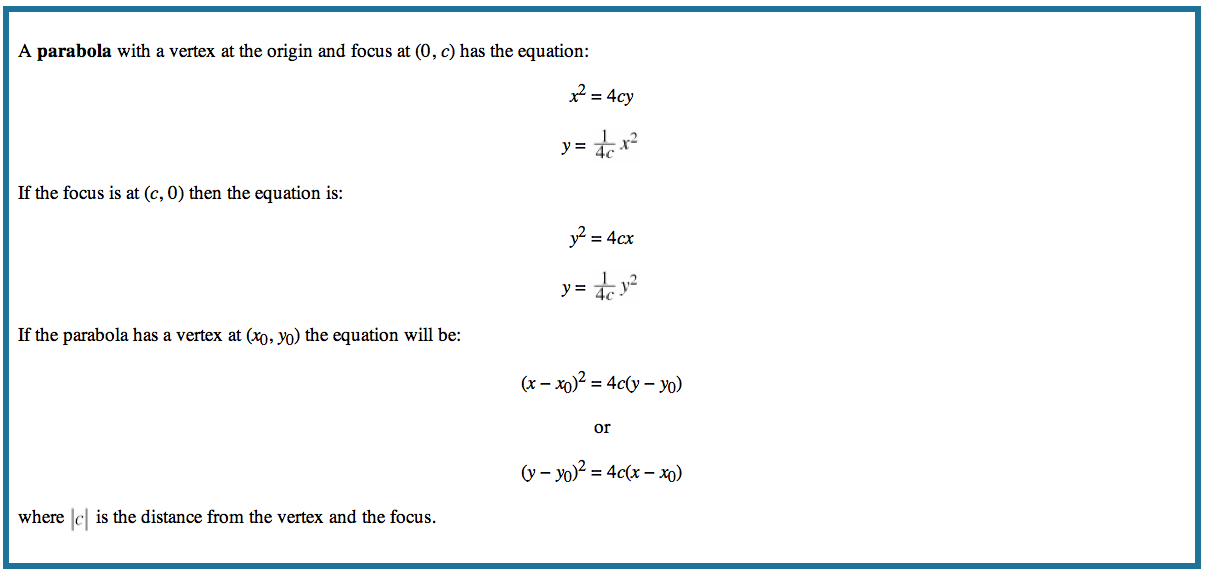13P-1.

### Missing problem for Book PCT => Chapter Ch13 => Lesson 13.1.4 => Problem 13P-1

Created from orphaned homework help problemIf 12 = 4c in this equation, what is c?

c = 3
The focus, therefore, is (0, 3)